# Monopulse Comparator Networks

New for October 2005! The comparator network (also known as an "arithmetic network") is what processes the four quadrants of a monopulse antenna into the SUM, Delta AZ, Delta EL, and Delta Q signals.

A monopulse antenna has four feeds, which can be horns or other radiators. We'll use the convention of the Cartesian coordinate system, where the quadrants are labeled starting in the upper right, then go counterclockwise, in this case,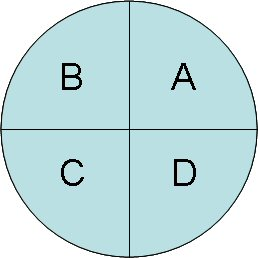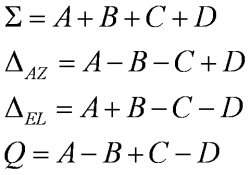Monopulse comparators can be made with either 180 degree or 90 degree hybrid couplers. We'll show you both ways!

### Monopulse comparators using rat-race hybrids

Below is a schematic representation of a monopulse comparator using 180 degree rat-race couplers. We've labeled the signals as they go through the network, so you can follow the arithmetic. You could try to find a better explanation of this important antenna concept somewhere else on the web, but you never will!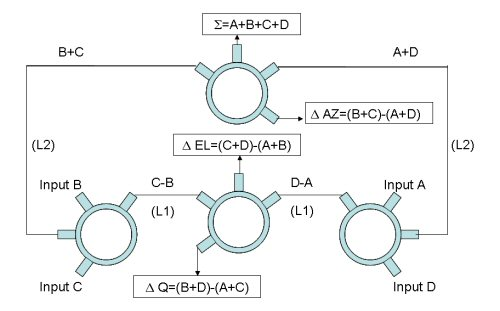Check it out, someone stole this figure and published it in Microwave Journal article!

The lengths of lines labeled "(L1)" don't matter, but they must remain the same phase. The same goes for "(L2)" lines. With all monopulse comparators, anything that introduces phase or amplitude errors on the four inputs is going to kill the response. So when you are laying this out, pay attention to every little detail, make the four inputs EXACTLY the same, and keep as much symmetry as possible.

We used Eagleware's Genesys program to simulate a rat-race comparator network, including response versus azimuth angle. We found it slightly awkward to use, especially in perfoming the comparator circuit math, which ideally is done using vectors but Eagleware insists that you first convert everything to real and imaginary parts first.

Our circuit model used a 50 um GaAs substrate (Er=12.9). We used 1 mm for the quarter-wavelength, which works out to a center frequency of about 27.6 GHz. Below is the schematic of the rat-race hybrid that we generated in Genesys: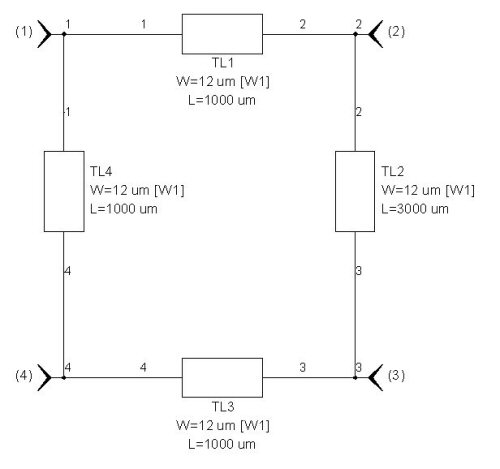The plots below shows some of the S-parameter frequency responses of this four-port rat-race network, in this case, the magnitude of the transmission between port 1 and the other three ports. Note that port 1 is the "sum" port, which means that a signal into this port splits equally to in-phase signals to ports 2 and 4. Port 3 is the "difference" port, which would also split equally into two signals at ports 2 and 4, but they would be anti-phase (180 degree difference).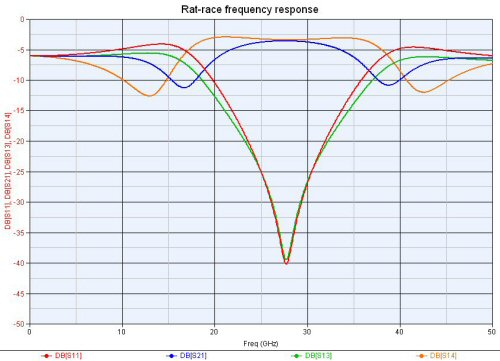Now it's time to build the comparator network. We know that we need a better figure below. Ports 1, 2, 3 and 4 are the antenna quadrant inputs A, B, C and D. The transmission lines TL1, TL2, and TL3 are used to add phase when the monopulse is tipped off of boresight (we'll explan this below). Following the arithmetic of the rat-race, signals at ports 2 and 4 are summed at port 1, and subtracted at port 3, for hybrid network N2 (top left). Etc.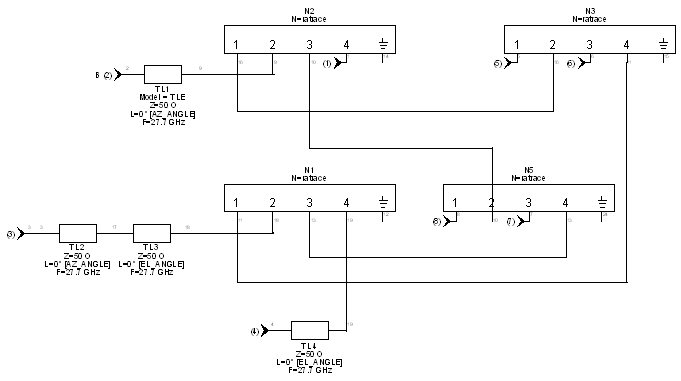Here are the equations we came up with to compute the sum and deltas responses using Genesys:

SUM=20*LOG((ABS(.re[s15]+Re[s25]+Re[s35]+Re[s45])^2+ABS(.IM[s15]+.IM[s25]+.IM[s35]+.IM[s45])^2)^0.5/2)

Delta_EL=20*LOG((ABS(Re[s16]+Re[s26]+Re[s36]+Re[s46])^2+ABS(.IM[s16]+.IM[s26]+.IM[s36]+.IM[s46])^2)^0.5/2)

Delta_Q=20*LOG((ABS(Re[s17]+Re[s27]+Re[s37]+Re[s47])^2+ABS(.IM[s17]+.IM[s27]+.IM[s37]+.IM[s47])^2)^0.5/2)

Delta_AZ=20*LOG((ABS(Re[s18]+Re[s28]+Re[s38]+Re[s48])^2+ABS(.IM[s18]+.IM[s28]+.IM[s38]+.IM[s48])^2)^0.5/2)

Yikes, that was painful!

Here are the transmission coefficients of signals into the four antenna quadrants, out the sum port.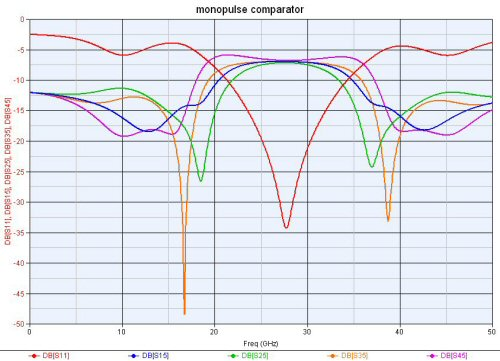Now let's look at the phases of the signals, and they exit the SUM, Delta AZ, Delta EL and Delta Q ports. The SUM port has all signals nearly in phase: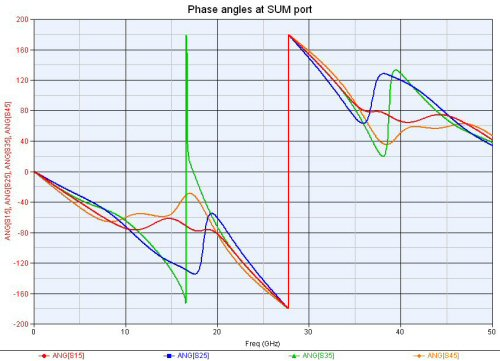The AZ port subtracts the left signals (ports 2 and 3) from the right (ports 1 and 4):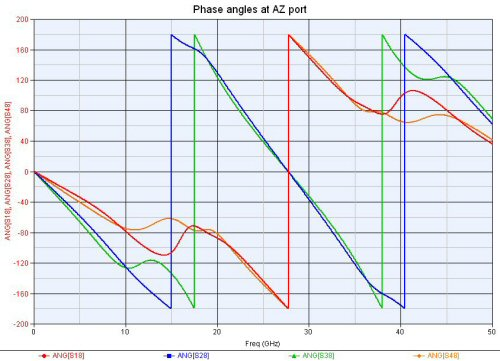The EL signal subtracts the top signals (1 and 2) from the bottom (3 and 4):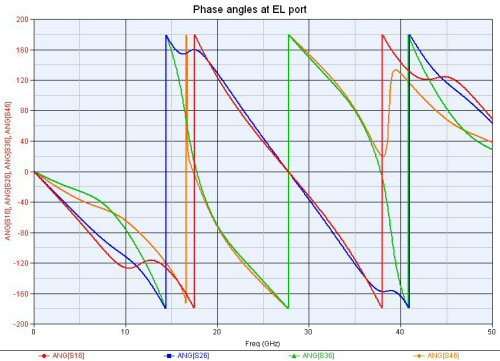We didn't plot the Q-channel but it subtracts diagonally. Typically this output is not used and just terminated.

Here are the sum and difference channels versus frequency: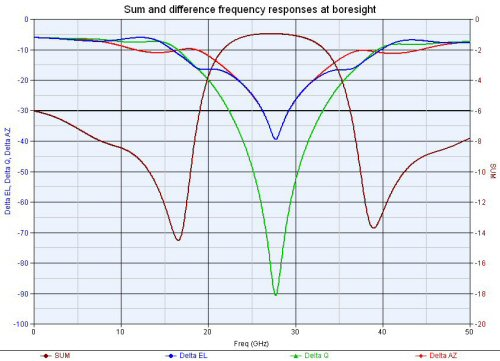Here are SUM and AZ versus added phase in the AZ plane, at center frequency: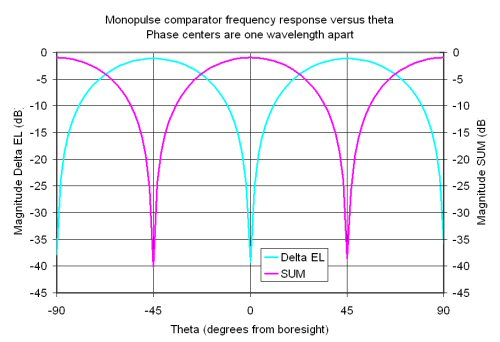### Monopulse comparator using 90 degree hybrids

Below is a schematic representation of a monopulse using 90 degree hybrid couplers, in this case, branchline couplers. The trick is to add a 90 degree section to one of the inputs of the coupler, converting it to a 180 degree coupler (click here to learn more). The layout has an advantage over the ratrace comparator in that none of the signals are "trapped" inside the network. The disadvantage is the bandwidth of this approach sucks!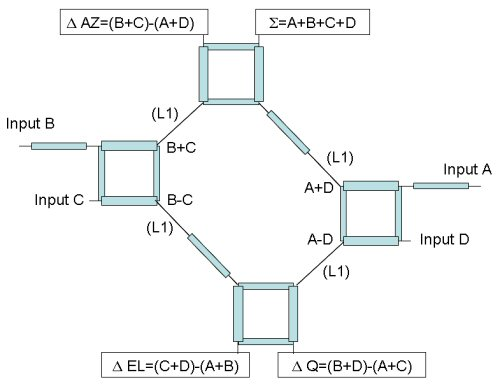In this comparator, all of the blue lines are quarterwavelength. The lengths of the four skinny black lines labeled "(L1)" don't matter much, but they all must be the same. Again, use care when you lay this out, errors of a few mils can mess up the response.

We didn't simulate the branchline coupler comparator, but if we get bored one of these days we will!

Author : Unknown Editor